Courses

# Wall Shear Stress - 2 Civil Engineering (CE) Notes | EduRev

## Mechanical Engineering : Wall Shear Stress - 2 Civil Engineering (CE) Notes | EduRev

The document Wall Shear Stress - 2 Civil Engineering (CE) Notes | EduRev is a part of the Mechanical Engineering Course Fluid Mechanics.
All you need of Mechanical Engineering at this link: Mechanical Engineering

Seperation of Boundary Layer

• It has been observed that the flow is reversed at the vicinity of the wall under certain conditions.
• The phenomenon is termed as separation of boundary layer
• Separation takes place due to excessive momentum loss near the wall in a boundary layer trying to move downstream against increasing pressure, i.e.,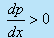, which is called adverse pressure gradient.
• Figure 29.2 shows the flow past a circular cylinder, in an infinite medium

1. Up to  θ = 900, the flow area is like a constricted passage and the flow behaviour is like that of a nozzle.
2. Beyond θ = 90 the flow area is diverged, therefore, the flow behaviour is much similar to a diffuser

• This dictates the inviscid pressure distribution on the cylinder which is shown by a firm line in Fig. 29.2.

Here

Pw   :  pressure in the free stream

U    :  velocity in the free stream and

P       : is the local pressure on the cylinder.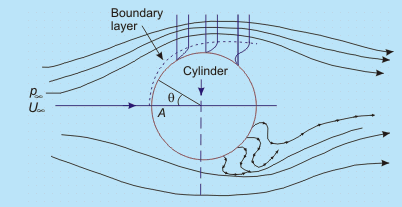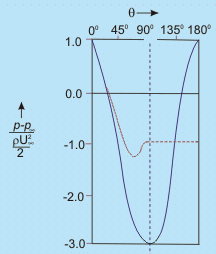Fig. 29.2   Flow separation and formation of wake behind a circular cylinder

1. Until θ = 900 the pressure force and the force due to streamwise acceleration i.e. inertia forces are acting in the same direction (pressure gradient beingnegative/favourable)
2. Beyond θ = 900 , the pressure gradient is positive or adverse. Due to the adverse pressure gradient the pressure force and the force due to acceleration will be opposing each other in the in viscid zone of this part.

So long as no viscous effect is considered, the situation does not cause any sensation.
In the viscid region (near the solid boundary),

1. Up to θ = 900 , the viscous force opposes the combined pressure force and the force due to acceleration. Fluid particles overcome this viscous resistance
due to continuous conversion of pressure force into kinetic energy.
2. Beyond θ = 900 , within the viscous zone, the flow structure becomes different. It is seen that the force due to acceleration is opposed by both the viscous force and pressure force.

• Depending upon the magnitude of adverse pressure gradient, somewhere around θ = 900the fluid particles, in the boundary layer are separated from the wall and driven in the upstream direction. However, the far field external stream pushes back these separated layers together with it and develops a broad pulsating wake behind the cylinder.
• The mathematical explanation of flow-separation : The point of separation may be defined as the limit between forward and reverse flow in the layer very close to the wall, i.e., at the point of separation( 29.16)

This means that the shear stress at the wall, tw = 0 . But at this point, the adverse pressure continues to exist and at the downstream of this point the flow acts in a reverse direction resulting in a back flow.

• We can also explain flow separation using the argument about the second derivative of velocity u at the wall. From the dimensional form of the momentum  at the wall, where u = v = 0, we can write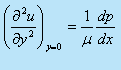( 29.17)

• Consider the situation due to a favourable pressure gradient where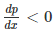we have,

1.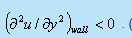. (From Eq. (29.17))
2. As we proceed towards the free stream, the velocity u approaches  U asymptotically, so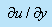decreases at a continuously lesser rate in y direction.
3.  This means that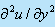remains less than zero near the edge of the boundary layer.
4.  The curvature of a velocity profileis always negative as shown in (Fig. 29.3a)
•      Consider the case of adverse pressure gradient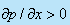1. At the boundary, the curvature of the profile must be positive (since).
2. Near the interface of boundary layer and free stream the previous argument regardingandstill holds good and the curvature is negative.
3.  Thus we observe that for an adverse pressure gradient, there must exist a point for which= 0 . This point is known as point of inflection of the velocity profile in the boundary layer as shown in Fig. 29.3b
4. However, point of separation means= 0 at the wall.
5.at the wall since separation can only occur due to adverse pressure gradient. But we have already seen that at the edge of the boundary layer,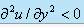. It is therefore, clear that if there is a point of separation, there must exist a point of inflection in the velocity profile.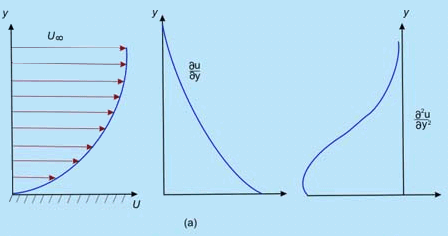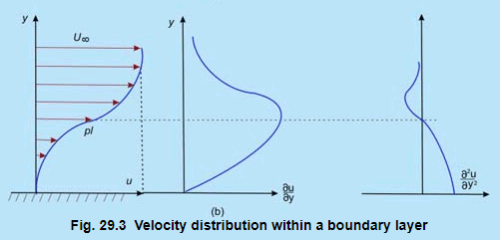(a) Favourable pressure gradient,(b) adverse pressure gradient,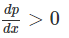1. Let us reconsider the flow past a circular cylinder and continue our discussion on the wake behind a cylinder. The pressure distribution which was shown by the firm line in Fig. 21.5 is obtained from the potential flow theory. However. somewhere near θ = 900 (in experiments it has been observed to be at θ = 810) . the boundary layer detaches itself from the wall.
2. Meanwhile, pressure in the wake remains close to separation-point-pressure since the eddies (formed as a consequence of the retarded layers being carried together with the upper layer through the action of shear) cannot convert rotational kinetic energy into pressure head. The actual pressure distribution is shown by the dotted line in Fig. 29.3.
3. Since the wake zone pressure is less than that of the forward stagnation point (pressure at point A in Fig. 29.3), the cylinder experiences a drag force which is basically attributed to the pressure difference.

The drag force, brought about by the pressure difference is known as form drag whereas the shear stress at the wall gives rise to skin friction dragGenerally, these two drag forces together are responsible for resultant drag on a body

Offer running on EduRev: Apply code STAYHOME200 to get INR 200 off on our premium plan EduRev Infinity!

## Fluid Mechanics

47 videos|82 docs|73 tests

,

,

,

,

,

,

,

,

,

,

,

,

,

,

,

,

,

,

,

,

,

;